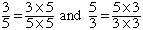how do I simplify radicals with fractional radicands? ex. 7sqrt(2) + sqrt(50) - 2sqrt(18) 10sqrt(3/5) - 24sqrt(5/3) 3sqrt(2/9) + 1/2sqrt(32) + sqrt(9/8) Hi, This is an exercise in recognizing numbers. When I look at the first problem what I see is that 50 = 252 and 18 = 92. That is, each of them is a square times 2. Thus sqrt(50) = sqrt(252) = 5sqrt(2) and sqrt(18) = sqrt(92) = 3sqrt(2) In the second problem I would like the denominators to be squares so that I can rewrite the expression with no fractions under the square root symbol. I can do this if I writeFor the third problem the key is 32 since 32 = 162, again a square times 2. Can you write each of the other two radicands as a square times 2? Penny Go to Math Central To return to the previous page use your browser's back button.